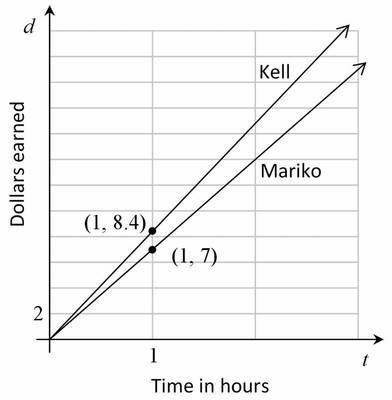# Who Has the Best Job?

Alignments to Content Standards: 8.EE.B.5

Kell works at an after-school program at an elementary school. The table below shows how much money he earned every day last week.

 Monday Wednesday Friday Time worked 1.5 hours 2.5 hours 4 hours Money earned \$12.60 \$21.00 \$33.60 Mariko has a job mowing lawns that pays \$7 per hour.

1. Who would make more money for working 10 hours? Explain or show work.

2. Draw a graph that represents $y$, the amount of money Kell would make for working $x$ hours, assuming he made the same hourly rate he was making last week.

3. Using the same coordinate axes, draw a graph that represents $y$, the amount of money Mariko would make for working $x$ hours.

4. How can you see who makes more per hour just by looking at the graphs? Explain.

## Solution

1. Mariko would make $7\times 10 = 70$ dollars for working 10 hours. Kell's hourly rate can be found by dividing the money earned by the hours worked each day.

 Monday Wednesday Friday Time worked 1.5 hours 2.5 hours 4 hours Money earned \$12.60 \$21.00 \$33.60 Pay rate \$8.40 per hour \$8.40 per hour \$8.40 per hour
If Kell works for 10 hours at this same rate, he will earn $8.4\times 10 = 84$ dollars. So Kell will earn more money for working 10 hours.

Alternatively, we could reason proportionally without computing the unit rate.  Since Mariko earned \$21.00 for 2.5 hours, she will earn four times as much for working four times as long ($10=4\times 2.5$), for a total of$4\times \$21=\$84$. 2. See the figure below. 3. See the figure below. 4. You can see that Kell will make more per hour if you look at the points on the graph where$x=1\$ since this will tell you how much money each person will make for working 1 hour. You can also compare the slopes of the two graphs, which are equal to the hourly rates. See the figure below.